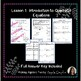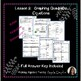Algebra Quadratic Equations Lesson Notes Practice Homework Quiz & TestSubject
Resource Type
Product Rating
4.0
2 Ratings
File Type

Compressed Zip File

18 MB|49 pages
Share
Also included in:
1. This is an entire curriculum for Algebra 1. It includes 12 units of individual lessons with full examples shown along with practice problems, homework and an editable quiz and test.All lessons are 100% prep free and with included answer keys make it easy for students who were absent to catch up.Incl
\$358.00
\$180.00
Save \$178.00
2. These materials are valued at over \$700. Purchase as a bundle and save big!! This is an entire curriculum for Algebra 1. It includes 12 units of individual lessons with full examples shown along with practice problems, homework and an editable quiz and test. Each unit includes games related to th
\$572.50
\$290.00
Save \$282.50
3. These materials are valued at over \$350. Purchase as a bundle and save big!! This is the second half of my curriculum for Algebra 1 and it will continue to GROW. It includes 6 units of individual lessons with full examples shown along with practice problems, homework, study guides, and 2 editable
\$288.49
\$145.00
Save \$143.49
4. These materials are valued at over \$350. Purchase as a bundle and save big!! This is the second half of my curriculum for Algebra 1 and it will continue to GROW. It includes 6 units of individual lessons with full examples shown along with practice problems, homework, study guides, and 2 editable
\$200.99
\$110.00
Save \$90.99
Product Description

This Item is part of my Algebra 1 curriculum BUNDLE with growing GAME PACK.

This is an entire unit on Quadratic Equations. It includes 5 lessons with full examples shown along with practice problems, homework, cumulative reviews after every lesson, and a quiz and test for this unit and (2) editable quizzes and tests for this unit.

All lessons are 100% prep free and with included answer keys make it easy for students who were absent to catch up.

Included Lessons:

Lesson 1 Introduction to Quadratic Equations

Lesson 3 Quadratic Equations in Vertex Form

Lesson 4 Solving Quadratic Equations by Graphing

Lesson 5 Solving Quadratic Equations by Factoring

Lesson 6 Solving Quadratic Equations by Finding Square Roots

2 Editable Tests and Quizzes

Interested in a fun interactive game covering all lessons in this unit? Check out

Prize Wheel Carnival Game Algebra Quadratic Equations PREP FREE

Here are some other units I have available:

Algebra Expressions & The Real Number System Lessons Notes Homework Quiz & Test

Algebra Equations & Inequalities Lesson Notes Homework Quiz & Test

Algebra Relations & Functions Lesson Notes Homework Quiz & Test

Algebra Slope & Writing Equations of Lines Lesson Notes Homework Quiz & Test

Algebra Systems of Equations Lesson Notes Practice Homework & Quiz

Exponent Rules with Monomials Lesson Notes BUNDLE Homework Quiz & Test

Algebra Polynomials BUNDLE Lesson Notes Practice Homework Quiz & Test

Algebra Factoring Polynomials BUNDLE Lesson Notes Practice Homework Quiz & Test

Algebra Quadratic Equations BUNDLE Lesson Notes Practice Homework Quiz & Test

Algebra Rational Expressions & Equation Lesson Notes Homework Quiz & Test

Algebra Radical Expressions & Equation Lesson Notes Homework Quiz & Test

Algebra Probability & Statistics 10 Lessons Notes Homework Quiz & Test BUNDLE

Don't miss my entire curriculum where you will receive all of these units for a BUNDLE price.

Algebra 1 curriculum BUNDLE Lesson Notes Homework Study Guides Quizzes & Tests

Prefer the curriculum with GROWING GAME PACK?

Algebra 1 curriculum BUNDLE with growing GAME PACK

Check out Making Algebra Fun for individual games.

The purchase of this product entitles you to personal use within one classroom. Sharing among colleagues is not permitted. Posting online or selling is strictly prohibited. Thank you for respecting my time and work.

Total Pages
49 pages